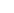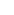# Maths ResourcesBrowse ResourcesTutor Hunt Resources

Why is financial literacy so important and why should we be teaching-learning about this at school!Few of us are prepared or are confident enough to discuss real-life money matters. l...

### What is the order of operations in maths?

Students in Year 6 and entrants for the 11+ exam need to know about the particular order in which to carry out mathematical calculations. Without this knowledge, it is easy to achieve...

### Is the Concrete Pictorial Abstract approach the most effect form of pedagogy?

Across schools in England, mathematics is taught using a ‘Concrete, Pictorial, Abstract’ (CPA) approach. The CPA approach is a technique where- The first stage of teaching is to ...### Effective Learning Strategies for Effectively Mastering Mathematics

Effective Learning Strategies for Effectively Mastering Mathematics ;IntroductionThe study of mathematics is both intriguing and difficult. While some people might like sol...

### Maths compulsory to 18?

In January 2023, UK Prime Minister Rishi Sunak announced plans to make the study of maths compulsory to age 18. In the UK, maths is already compulsory up to the age of 16. Another two...

### Exam Year

How do we get the results we think we deserve?With mocks fast approaching, and exams around the corner, it can feel like time is running out. Breathe.The start of the exam year is a g...

### Quicker root 2 irrationality proof.

Firstly, a rational number is any number expressible as the quotient of 2 integers, hence the group of rational numbers is labelled by the letter `Q`. E.g., 5 = 10/2, among many more ...

### Why is the derivative of sinx = cosx?

The derivative of any function f(x) can be found from first principles as the difference between two values of f(x) infinitely close to each other divided by the infinitesimal differe...### Oxbridge Interview Question

Sample question:Here is an example of a question which could come up in an Oxford interview. Normally, what happens is that the interviewer will begin with an easier question and then...### How do we find a formula for a simple sequence?

Here is a question:Find the 32nd term of the sequence 6, 11, 16, 21.... 1. Find the difference between the numbers So 11-6 or 21-16 will give 52. 5 is the difference so we have the ...
See All ResourcesView All Subjects